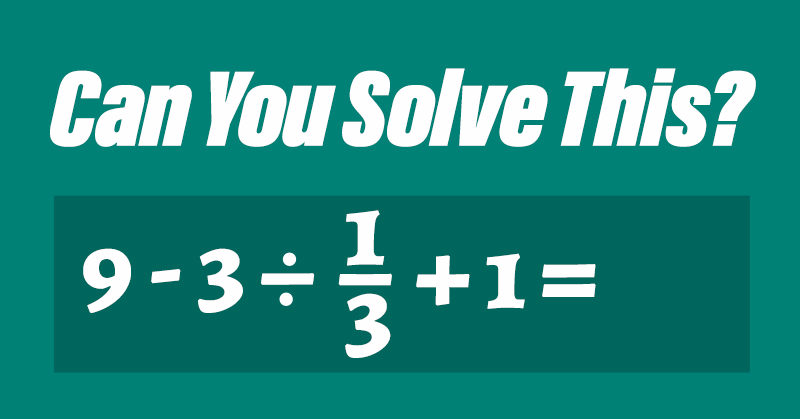Julie Hambleton
October 19, 2020 · 1 min read

# Can You Solve This Math Problem That Went Viral In Japan?

A new math problem went viral in Japan because of how many people were unable to solve it. This Japanese math problem has made its way across the ocean and now we’re challenging you to see if you can solve it.

## The Viral Japanese Math Problem

In Japan in the 1980s, 90% of 20-year-olds were able to solve this math problem. Now only 60% of people in that same age group were able to solve it. (1)

Can you solve this Japanese math problem?

## A Trick to Remember

It has been a long time since many of us have had to do math without using a calculator. The thing is, if you write this Japanese equation into your smartphone calculator, you will most likely be given the wrong answer.
This is why many of us have forgotten some of the basic principles of solving equations that we learned in school.

To solve this Japanese math problem, you have to remember the Order of Operations. The simple way to do this is by using the acronym BEDMAS:

B – brackets
E – exponents
D – division
M – multiplications